# 多柔体车辆耦合系统对动力学性能的影响分析Analysis of the Influence of Multi-Flexible Vehicle Coupling System on Dynamic Performance

DOI: 10.12677/OJTT.2021.101001, PDF, HTML, XML, 下载: 88  浏览: 175

Abstract: In order to study the influence of the rigid-flexible coupling vehicle system on the dynamic performance of the railway vehicle in the running state, this paper takes a high-speed EMU trailer as the research object, and builds the rigid-flexible coupling vehicle model based on SIMPACK multi-body dynamics software and the rigid-flexible coupling vehicle model coupled by flexible wheel set, flexible frame and flexible body structure. In order to evaluate the influence of rigid-flexible coupling modeling on the dynamic performance, the results of simulation calculation are compared in different operating speeds and different operating conditions. The results show that compared with the calculation results of multi rigid vehicle, the rigid-flexible coupling vehicle as a whole shows poor performance in stability, running quality and curve passing performance, especially in the high-speed running state, the vertical impact of rigid flexible coupling vehicle is more severe.

1. 前言

2. 车辆系统动力学相关指标

2.1. 车辆运行稳定性指标

2.2. 车辆运行平稳性指标

$W=0.896\sqrt{\frac{{a}^{3}}{f}\cdot F\left(f\right)}$ (1)

$F\left(f\right)=\left\{\begin{array}{l}0.325\cdot {f}^{2}\text{\hspace{0.17em}}\text{\hspace{0.17em}}\text{\hspace{0.17em}}\left(f=0.5~5.9\text{\hspace{0.17em}}\text{Hz}\right)\\ 400/{f}^{2}\text{\hspace{0.17em}}\text{\hspace{0.17em}}\text{\hspace{0.17em}}\text{\hspace{0.17em}}\text{\hspace{0.17em}}\text{ }\text{\hspace{0.17em}}\left(f=5.9~20\text{\hspace{0.17em}}\text{Hz}\right)\\ 1\text{\hspace{0.17em}}\text{\hspace{0.17em}}\text{\hspace{0.17em}}\text{\hspace{0.17em}}\text{\hspace{0.17em}}\text{\hspace{0.17em}}\text{\hspace{0.17em}}\text{\hspace{0.17em}}\text{\hspace{0.17em}}\text{\hspace{0.17em}}\text{\hspace{0.17em}}\text{\hspace{0.17em}}\text{\hspace{0.17em}}\text{\hspace{0.17em}}\text{\hspace{0.17em}}\text{\hspace{0.17em}}\text{\hspace{0.17em}}\text{\hspace{0.17em}}\text{\hspace{0.17em}}\text{\hspace{0.17em}}\text{\hspace{0.17em}}\text{\hspace{0.17em}}\text{\hspace{0.17em}}\text{\hspace{0.17em}}\left(f>20Hz\right)\end{array}$ (2)

$F\left(f\right)=\left\{\begin{array}{l}0.8\cdot {f}^{2}\text{\hspace{0.17em}}\text{\hspace{0.17em}}\text{\hspace{0.17em}}\left(f=0.5~5.4\text{\hspace{0.17em}}\text{Hz}\right)\\ 650/{f}^{2}\text{\hspace{0.17em}}\text{\hspace{0.17em}}\text{\hspace{0.17em}}\left(f=5.4~26\text{\hspace{0.17em}}\text{Hz}\right)\\ 1\text{\hspace{0.17em}}\text{\hspace{0.17em}}\text{\hspace{0.17em}}\text{\hspace{0.17em}}\text{\hspace{0.17em}}\text{\hspace{0.17em}}\text{\hspace{0.17em}}\text{\hspace{0.17em}}\text{\hspace{0.17em}}\text{\hspace{0.17em}}\text{\hspace{0.17em}}\text{\hspace{0.17em}}\text{\hspace{0.17em}}\text{\hspace{0.17em}}\text{\hspace{0.17em}}\text{\hspace{0.17em}}\text{\hspace{0.17em}}\text{\hspace{0.17em}}\text{\hspace{0.17em}}\text{\hspace{0.17em}}\left(f>26\text{\hspace{0.17em}}\text{Hz}\right)\end{array}$ (3)Table 1. Evaluation criteria for running stability of passenger cars

2.3. 曲线通过性能

2.3.1. 脱轨系数

$\frac{{Q}_{1}}{{P}_{1}}=\frac{tg{\alpha }_{1}-{\mu }_{1}}{1+{\mu }_{1}tg{\alpha }_{1}}$ (4)

2.3.2. 英文作脱轨系数

$\left\{\begin{array}{c}\stackrel{¯}{P}=\frac{1}{2}\left({P}_{1}+{P}_{2}\right)\\ \Delta P=\frac{1}{2}\left({P}_{2}+{P}_{1}\right)\\ {P}_{1}=\stackrel{¯}{P}-\Delta P\\ {P}_{2}=\stackrel{¯}{P}+\Delta P\end{array}$ (5)

3. 车辆系统动力学建模

3.1. 多刚体车辆模型建立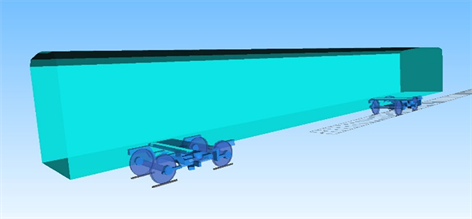Figure 1. Schematic diagram of multi-rigid vehicle model

3.2. 多刚体车辆柔性体模型建立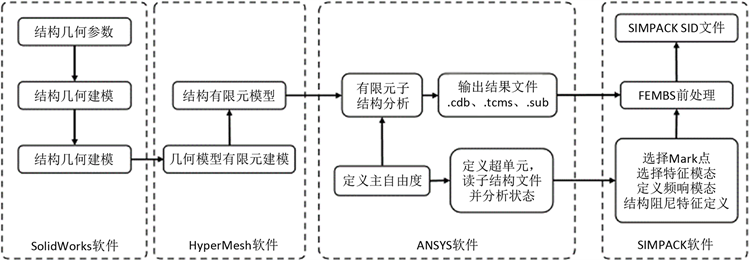Figure 2. The flexible structure modeling process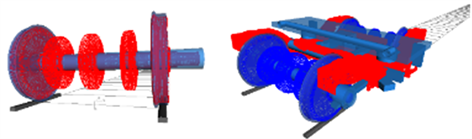(a) 柔性轮对结构(红) (b) 柔性构架结构(红)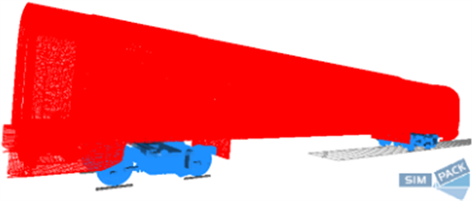(c) 杠头耦合车辆模型

Figure 3. Schematic diagram of rigid-flexible coupling vehicle model

3.3. 轨道参数

4. 仿真流程

5. 仿真计算结果分析

5.1. 临界速度

5.2. 车辆运行平稳性指标差异

5.2.1. 车体加速度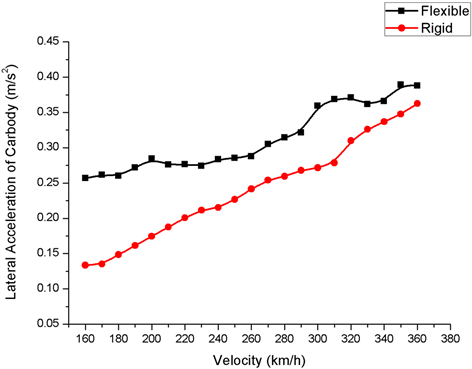Figure 4. Lateral acceleration of car body under straight running conditions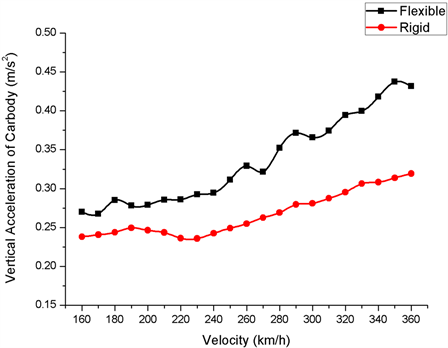Figure 5. Vertical acceleration of car body under straight-line running conditions

5.2.2. Sperling平稳性指标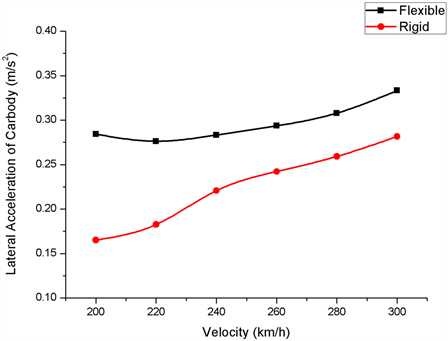Figure 6. Lateral acceleration of car body under curve running conditions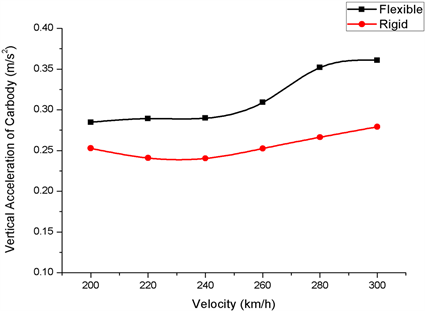Figure 7. Vertical acceleration of car body under curve running conditions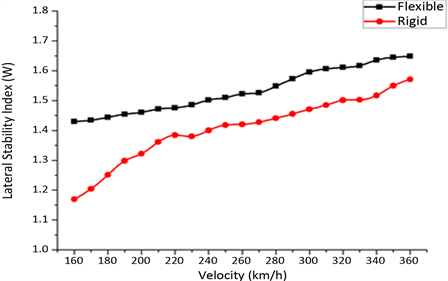Figure 8. Comparison of horizontal stability indicators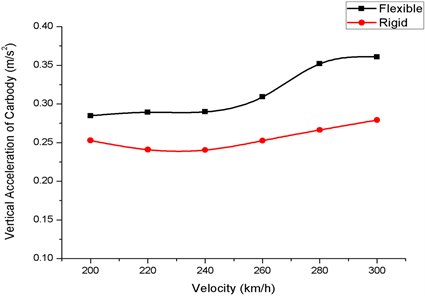Figure 9. Comparison of vertical stability indicators

5.3. 曲线通过性能分析

5.3.1. 脱轨系数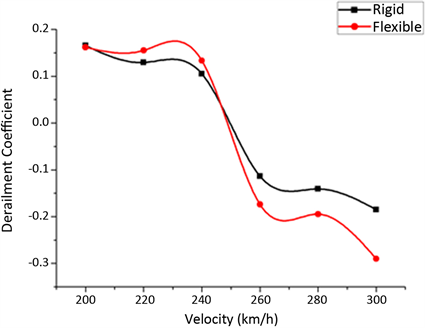Figure 10. Comparison of derailment coefficient indicators

5.3.2. 轮重减载率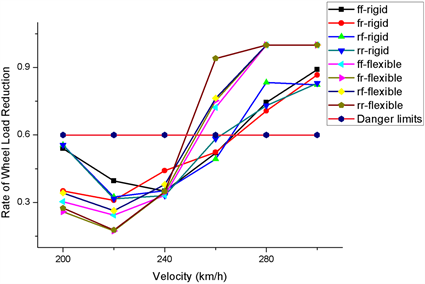Figure 11. Comparison of wheel load reduction rate index

6. 结论

1) 临界速度指标中，刚柔耦合车辆模型的临界速度相比于多刚体车辆模型降低了10.56%，采用多刚体车辆模型作为结果参考，会高估车辆在运行时刻的极限表现。

2) 在车体加速度、车辆运行品质以及舒适度指标中，刚柔耦合车辆模型仿真结果高于多刚体车辆模型，表明考虑结构的柔性变形和整体的耦合效应导致车辆平稳性指标的表现更加危险。

3) 车辆曲线通过性能指标中，刚柔耦合车辆模型相比于多刚体车辆模型仿真结果显示，刚柔耦合模型对于曲线通过能力欠佳，尤其运行速度在260 km/h以上时，两者曲线通过性能的差异随速度的增加呈现逐渐增大的趋势。

  徐凯, 李芾, 安琪. 车辆系统刚柔耦合动力学方法研究[J]. 机车电传动, 2014(6): 24-27+30.  郭训, 郑树彬, 柴晓冬, 李立明. 基于柔性轮对的轨道车辆动力学仿真分析[J]. 测控技术, 2018, 37(8): 150-153.  吴少培, 赵泽福, 姚永明, 李国芳, 丁旺才. 构架柔性对车辆振动特性的影响[J]. 兰州交通大学学报, 2020, 39(1): 80-87+100.  杨丹丹. 基于SIMPACK的某型列车整车刚柔耦合建模及模型修正[D]: [硕士学位论文]. 南京: 南京航空航天大学, 2016.  Popp, K., Kaiser, I. and Kruse, H. (2003) System Dynamics of Railway Vehicles and Track. Archive of Applied Mechanics, 72, 949-961. https://doi.org/10.1007/s00419-002-0261-6  严隽耄, 傅茂海. 车辆工程[M]. 北京: 中国铁道出版社, 2008.  Gargvk, Dukkipatirv, 沈利人. 铁道车辆系统动力学[M]. 成都: 西南交通大学出版社, 1998.  周素霞, 等. SIMPACK9实例教程(下) [M]. 北京: 北京联合出版公司, 2013.  任尊松. 车辆系统动力学[M]. 北京: 中国铁道出版社, 2007: 144-147.  贺建功. 轮对柔性对车辆系统动力学性能的影响[D]: [硕士学位论文]. 成都: 西南交通大学, 2011.  张孟, 张轮, 罗意平, 等. 高速动车转向架动力学性能SIMPACK仿真建模与分析[J]. 城市轨道交通研究, 2015, 46-51.